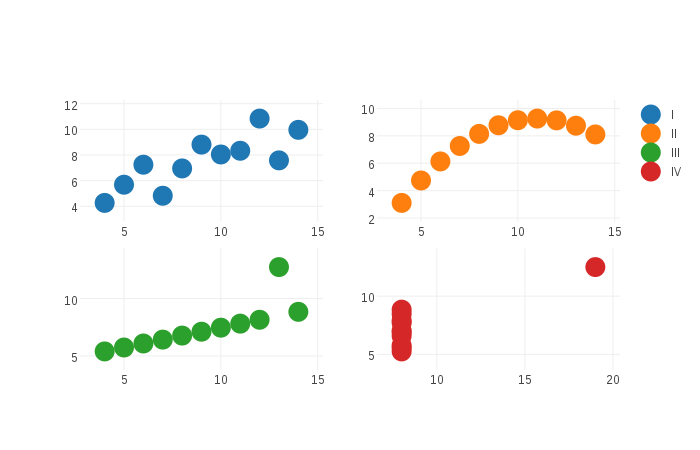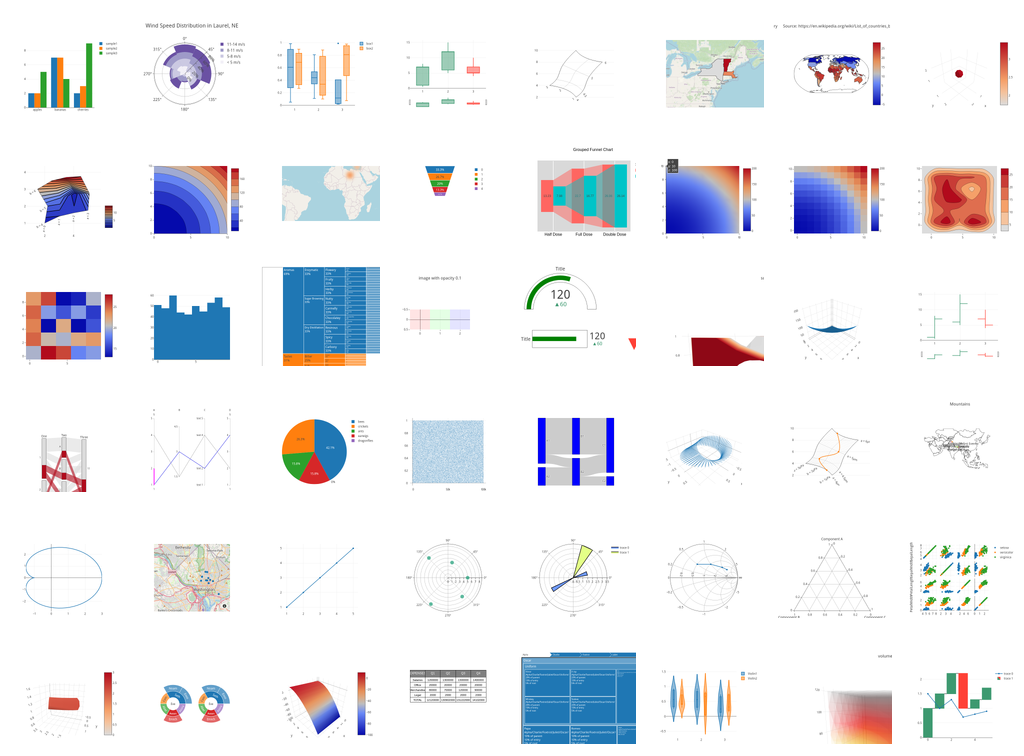and 2 contributors

# NAME

Chart::Plotly - Generate html/javascript charts from perl data using javascript library plotly.js

version 0.030

# SYNOPSIS

`````` use Chart::Plotly 'show_plot';

my \$data = { x    => [ 1 .. 10 ],
mode => 'markers',
type => 'scatter'
};
\$data->{'y'} = [ map { rand 10 } @{ \$data->{'x'} } ];

show_plot([\$data]);

use aliased 'Chart::Plotly::Trace::Scattergl';

my \$big_array = [ 1 .. 10000 ];
my \$scattergl = Scattergl->new( x => \$big_array, y => [ map { rand 100 } @\$big_array ] );

show_plot([\$scattergl]);

use Chart::Plotly qw(show_plot);
use PDL;

use aliased 'Chart::Plotly::Trace::Surface';

my \$size = 25;
my \$x    = ( xvals zeroes \$size+ 1, \$size + 1 ) / \$size;
my \$y    = ( yvals zeroes \$size+ 1, \$size + 1 ) / \$size;
my \$z    = 0.5 + 0.5 * ( sin( \$x * 6.3 ) * sin( \$y * 6.3 ) )**3;    # Bumps

my \$surface = Surface->new( x => \$x, y => \$y, z => \$z );

show_plot([\$surface]);

use PDL::Math;

my \$bessel_size = 50;
my \$bessel      = Surface->new(
x => xvals(\$bessel_size),
y => xvals(\$bessel_size),
z => bessj0( rvals( zeroes( \$bessel_size, \$bessel_size ) ) / 2 )
);

show_plot([\$bessel]);``````

# DESCRIPTION

Generate html/javascript charts from perl data using javascript library plotly.js. The result is a file that you could see in your favourite browser.

Example screenshot of plot generated with examples/anscombe.pl:Example screenshot of plots generated with examples/traces/*.pl:The API is subject to changes.

# FUNCTIONS

## render_full_html

### Parameters

• data:

Data to be represented. It could be:

Perl data structure of the json expected by plotly.js: http://plot.ly/javascript/reference/ (this data would be serialized to JSON)
Array ref of objects of type Chart::Plotly::Trace::*
Anything that could be serialized to JSON with the json expected by plotly.js

## html_plot

Return the html for the plot or plots

### Parameters

Data to be represented. The format is the same as the parameter data in render_full_html. Accepts multiple traces/plots/objects.

## show_plot

Opens the plot or plots in a browser locally

### Parameters

Data to be represented. The format is the same as the parameter data in render_full_html. Accepts multiple traces/plots/objects.

## plotlyjs_version

Returns the version of plotly.js using in this version of the perl module as a string

# BUGS

Please report any bugs or feature requests via github: https://github.com/pablrod/p5-Chart-Plotly/issues

# DISCLAIMER

This is an unofficial Plotly Perl module. Currently I'm not affiliated in any way with Plotly. But I think plotly.js is a great library and I want to use it with perl.

If you like plotly.js please consider supporting them purchasing a pro subscription: https://plot.ly/products/cloud/

# AUTHOR

Pablo Rodríguez González <pablo.rodriguez.gonzalez@gmail.com>

``  The MIT (X11) License``